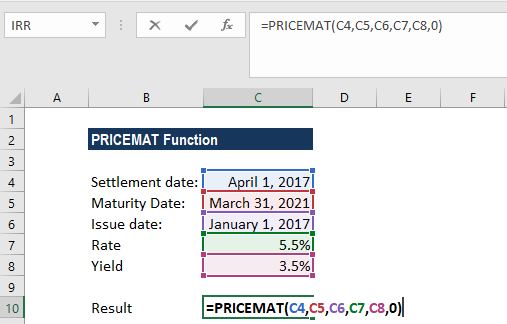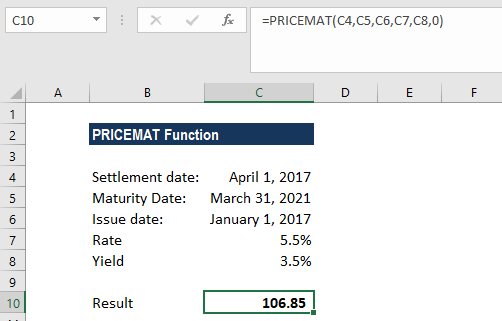# PRICEMAT Function

Calculates the price of a bond that pays interest at maturity per \$100 face value

## What is the PRICEMAT Function?

The PRICEMAT Function is categorized under Excel FINANCIAL functions. It will calculate the price of a bond that pays interest at maturity, per \$100 face value.

In financial analysis, the PRICEMAT function can be useful when we wish to borrow money by selling bonds instead of stocks. We need to know the minimum figure to charge investors. We can calculate its price per \$100 of redemption value using PRICEMAT.

### Formula

=PRICEMAT(settlement, maturity, issue, rate, yld, [basis])

The PRICEMAT function uses the following arguments:

1. Settlement (required argument) – The security’s settlement date or the date that the coupon is purchased. The security’s settlement date should be after the issue date.
2. Maturity (required argument) – This is the security’s maturity date or the date when the security expires.
3. Issue (required argument) – The security’s issue date that is expressed as a serial date number.
4. Rate (required argument) – This is the interest rate on the security on the date of issue.
5. Yld (required argument) – This is the annual yield of the security.
6. Basis (optional argument) – Specifies the financial day count basis that is used by the security.

BasisDay Count basis
0 or omittedUS(NASD) 30/360
1Actual/actual
2Actual/360
3Actual/365
4European 30/360

### How to use the PRICEMAT Function in Excel?

As a worksheet function, PRICEMAT can be entered as part of a formula in a cell of a worksheet. To understand the uses of the function, let’s consider an example:

#### Example

If we wish to calculate the price per \$100 face value of a security that pays interest at maturity, we can do it using the PRICEMAT function. The security’s issue date is January 1, 2017, the settlement date is April 1, 2017, and the maturity date is March 31, 2021. The rate of interest at issue is 5.5% and the annual yield is 3.5%. The US (NASD) 30/360 day count basis is used. We provide references to the cells in the formula.We get the result below:The PRICEMAT function returns the value 106.85 (rounded off to 2 decimal points). The price of the security with the above terms would be \$106.85. In the example above:

1. As recommended by Microsoft Excel, we provided as input the settlement, maturity, and issue dates as references to cells containing dates.
2. The rate and yld arguments are provided as input as percentages (5.5% and 3.5%, respectively). However, the arguments can instead be entered as the simple numerical values 0.055 and 0.035, respectively.
3. If the [basis] argument is omitted, the function will use the default value 0 (denoting the US (NADS) 30/360 day count basis). Here, we provided it as 0.

### A few notes about the PRICEMAT Function:

1. #NUM! error – Occurs if either:
1. The issue date is greater than or equal to the settlement date.
2. The settlement date is greater than or equal to the maturity date.
3. When we provide invalid numbers for the rate, yield, or basis arguments. That is, if we provided a rate that is less than zero, a yield that is less than zero, or basis is a number other than 0,1,2,3,4.
2. #VALUE! error – Occurs if:
1. The given settlement, maturity, or issue arguments are invalid Excel dates.
2. Any of the given arguments are non-numeric.

Thanks for reading CFI’s guide to the Excel PRICEMAT function. By taking the time to learn and master these functions, you’ll significantly speed up your financial analysis. To learn more, check out these additional CFI resources:

• Excel Functions for Finance